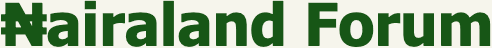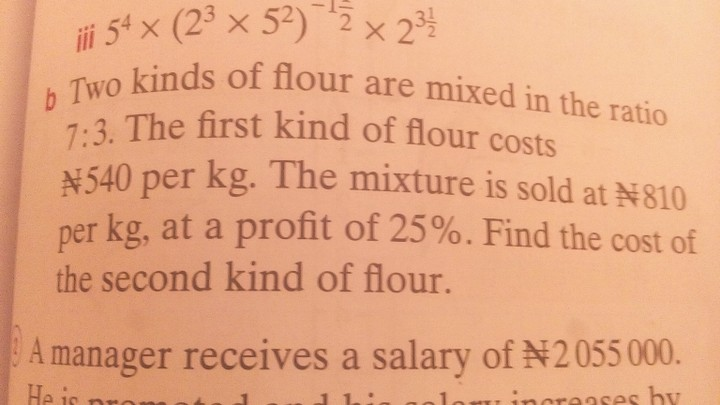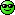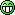#Welcome, Guest: Register On Nairaland / LOGIN! / Trending / Recent / New
Stats: 2,906,917 members, 7,008,762 topics. Date: Tuesday, 09 August 2022 at 10:03 PM

## Mathematics Problems - Education - Nairaland

 Mathematics Problems by monsieurbanky: 11:38am On Jan 26, 2018 I need someone genius to help me solve this mathematics problem that is giving me headache .thanksRe: Mathematics Problems by dejt4u(m): 1:32pm On Jan 26, 2018 N108 Re: Mathematics Problems by ameristu: 1:40pm On Jan 26, 2018 The second flour cost N796.30 per kg Re: Mathematics Problems by Sugar97(f): 3:25pm On Jan 26, 2018 Its N900 per kgSee workings:First you must remove the profit element from the selling price. This is easily done by dividing the selling price of the mixture (per kg) by 1.25 since the profit is 25% of cost. Now 810/1.25 = 648. This is the cost of the mixture per kg.Now you must form an equation as follows:7/10 (540) + 3/10 (x) = 648The first term of the equation represents the first kind of flour (recall that it costs 540 per kg)The second term of the equation represents the other kind of flour (the cost per kg is unknown hence x)The sum of both terms must give N648 which is the cost of the mixture.Finally solve the equation for x and you'll find that it is N900 per kg.Re: Mathematics Problems by monsieurbanky: 5:43pm On Jan 26, 2018 The answer is indeed 900.u are a genius. I really appreciate you .thanks .I can. Now go back to my students . Re: Mathematics Problems by Chuvin22(m): 7:33pm On Jan 26, 2018 Headache loading...